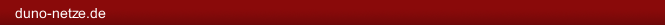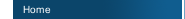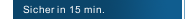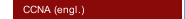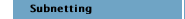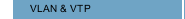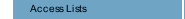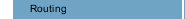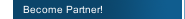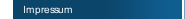When we write a Subnetmask in binary, we will see - no matter, which Subnetmask we use - that there are allways some "ones" followed by "zeros". For example

11111111.11111111.11111111.11100000 = 255.255.255.224

11111111.11111111.00000000.00000000 = 255.255.0.0

The number of the used "zeros" is a indicator, how many hosts can take place in that subnet, means how many IP-addresses are useable in that subnet for host-PCs.

If we take a look at the example network

192.168.10.0

255.255.255.0

We have here ONE network, with 254 useable IPs for Client-PCs.

If we write down the Subnetmask in binary, we will see why.

The Number 255 is in binary

11111111 = 128+64+32+16+8+4+2+1 = 255

The whole Subnetmask in the example is in binary

11111111.11111111.11111111.00000000

If we calculate all the 8 zeros as powers of 2, we will come to the result.

2^8=256

But - from this 256 IPs we must take one IP for the Broadcast-IP, and one for the Network-IP. So there are 254 IPs left, useable for Host-PCs in that Network.

This rule is also known as Formula

2^n-2

The IP Range of this network is

 First IP 192.168.10.1 Last IP 192.168.10.255

So we can give PCs in that network IPs like

 192.168.10.1 Host PC 1 192.168.10.2 Host PC 2 192.168.10.3 Host PC 3

and so on, till the end of the network is reached with 192.168.10.254.

If we want to divide this network in two parts, we need two things: a router and some switches - and of cause someone, who can calculate subnets.

With Subnetmask 255.255.255.128 we would divide the network in two parts.

192.168.10.1 - 192.168.10.127

192.168.10.128 - 192.168.10.254

The Subnetmask defines how big the subnet is. That means - how many Client-PCs will have place in that subnetwork.

A Subnetmask of 255.255.255.0 means in binary

11111111.11111111.11111111.0

So, what do we see?

4 Blocks, divided with a ".". Each of these blocks is also called "octett". Because - each Block has 8 bits.

To be able to do subnet-calculation, we first must understand binary calculation.

Lets take a deeper view at the first octett.

11111111

The first "1" stands for a 128.

The second "1" stands for a 64.

The third "1" stands for a 32.

The fourth "1" stands for a 16.

The fifth "1" stands for a 8.

And so on. That means:

11111111=255=128+64+32+16+8+4+2+1

11110000=240=128+64+32+16+0+0+0+0

11100000=224=128+64+32+0+0+0+0+0

If we see something like "/24", that means that 24 bits are set to "1", from the left side.

Examples:

/16 = 255.255.0.0 = 11111111.11111111.00000000.00000000

/20 = 255.255.240.0 = 11111111.11111111.11110000

Calculation of Subnetmask big enough for a specified number of Hosts

Question: "How to create a subnet with minimum 10 host IPs"

1. Step

calculate a power of two, that is minimum 10

2^3=8. That is not enough
2^4=16 That is higher than 10. Good.

2. Step

11111111.11111111.11111111.11110000

That is in decimal

255.255.255.240

With THIS Subnetmask, you have minimum 10 Host-Ips for each Subnet, without wasting to much IP-Addresses.

--------------------------------------------------------
Other example

"Create a subnet with minimum 70 Host-IPs"

1. Step

Calculate a Power of 2 that is MINIMUM 70

2^6=64. Not enough.
2^7=128. Thats higher than 70. Good.

2. Step

11111111.11111111.11111111.10000000

That is in decimal

255.255.255.128

You have a Subnet, with more than 70 Host-IPs.

Calculation what is the Broadcast-IP of a Subnet

"There is subnet 172.16.64.0/20. What is the BROADCAST ADDRESS of that Subnet?"

1. Step

/20 meens 255.255.240.0

2. Step

Now analyze the Subnet Oktett to find out the "network-jumps" or "increments".

240 means 11110000

The LAST of the 1s is under decimal 16. That are our "network jumps"
(128/64/32/16/8/4/2/1)

3. Step

Write down the network-jumps

172.16.64.0 - 172.16.79.255
+16 172.16.80.0 - 172.16.95.255
+16 172.16.96.0 - 172.16.111.255
+16 172.16.112.0 - 172.16.127.255

Because the NEXT Subnet in the example is 172.16.80.0, the broadcast must be 172.16.79.255, cause THAT is the IP BEFORE the next Subnet starts = the BroadcastAddress.

--------------------------------------------------------

If it would be 172.16.64.0 /26

Same procedure

/26 means 255.255.255.192

192 is binary 11000000
The LAST 1 stands under the 64.

That are in that example our "net-jumps".

172.16.64.0 - 172.16.64.63 <<<in this example THIS is the broadcastaddress of first subnet
172.16.64.64 - 172.16.64.127
172.16.64.128 - 172.16.64.191

Calculating first and last possible IP for a Host

Question: "You have Network 192.168.20.32 /27
The very first IP is reserved for Default Gateway!
What is the first and last valid IP for a Host-PC?"

1.Step

/27 is 255.255.255.224

2.Step

224 means 11100000

The LAST 1 is under the 32. That are our "network-jumps" in this example
(128/64/32/16/8/4/2/1)

Valid IPs in that subnet:
192.168.20.33 - 192.168.20.62

192.168.20.63 is NOT useable, this is the very last IP and so the BROADCAST-IP.
192.168.20.64 is the network-IP of the NEXT Subnet!

So, because the very first IP is reserved for Default Gateway, our first Host-PC IP would be
192.168.20.34
The very last Host-PC IP would be
192.168.20.62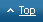duno-netze.de | infoduno-netze.de